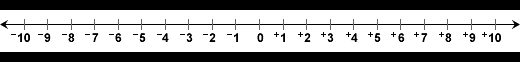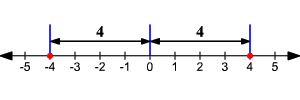Forum - - integers

# - integers

the lntegers

 The integers  are formed by the natural numbers (including 0) (0, 1, 2, 3, ...) together with the negatives of the non-zero natural numbers (−1, −2, −3, ...). , they are numbers that can be written without a fractional or decimal component, and fall within the set {..., −2, −1, 0, 1, 2, ...}. For example, 21, 4, and −2048 are integers; 9.75, 5½, are not integers.

the number linedistance from zerothe distance of  -3 from 0 is 3

the distance of 5 from 0 is    5

the opposite of numbre4 and -4  are opposlte numbers

 exercise write those numbers frome least to greatest             11 ,  -1  ,    0     ,      7      ;       - 12

exercises